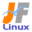Linux Kernel 2.6 Documentation: /usr/src/linux/Documentation/filesystems/ubifs.txt

# filesystems/ubifs.txt

UBIFS \$B\$N2r@b(B [\$B%W%l%\$%s%F%-%9%HHG(B]
• \$B86Cx:n
• \$BK]Lu
• \$B%P!<%8%g%s(B: 2.6.29
• \$BK]LuF|;~(B: 2009/03/25
```

=========================================================
\$B\$3\$l\$O!"(B
Linux-2.6.29/Documentation/filesystems/ubifs.txt \$B\$NOBLu(B
\$B\$G\$9!#(B
\$BK]LuCDBN!'(B JF \$B%W%m%8%'%/%H(B < http://www.linux.or.jp/JF/ >
\$B99?7F|(B \$B!'(B 2009/03/25
\$BK]Lue\$GF0\$/\$h\$&@_7W\$5\$l\$F\$\$\$^\$9!#M}(B
\$B2r\$N>e\$G=EMW\$JE@\$O!"(BUBIFS \$B\$O(B Linux \$B\$N=>Mh\$N%U%!%\$%k%7%9%F%`(B (\$BNc\$(\$P(B Ext2\$B!"(B
XFS\$B!"(BJFS \$BEy(B) \$B\$N\$\$\$:\$l\$H\$bA4\$/0[\$J\$k\$b\$N\$G\$"\$k\$H8@\$&\$3\$H\$G\$9!#(BUBIFS \$B\$O!"%V%m(B
\$B%C%/%G%P%\$%9\$G\$O\$J\$/(B MTD \$B%G%P%\$%9\$GF0\$/A4\$/JL\$N%U%!%\$%k%7%9%F%`\$N%/%i%9\$rBe(B
\$BI=\$7\$F\$\$\$^\$9!#\$3\$l0J30\$K\$3\$N%/%i%9\$KB0\$9\$k%U%!%\$%k%7%9%F%`\$K\$O(B JFFS2 \$B\$,\$"\$j(B
\$B\$^\$9!#(B

#To make it more clear, here is a small comparison of MTD devices and
#block devices.
\$B\$b\$&>/\$7L@3N\$K\$9\$k\$?\$a\$K!"0J2<\$G(B MTD \$B%G%P%\$%9\$H%V%m%C%/%G%P%\$%9\$N4JC1\$JHf3S(B
\$B\$r\$7\$^\$7\$g\$&!#(B

#1 MTD devices represent flash devices and they consist of eraseblocks of
#  rather large size, typically about 128KiB. Block devices consist of
#  small blocks, typically 512 bytes.
1 MTD \$B%G%P%\$%9\$O%U%i%C%7%e%G%P%\$%9\$KBP1~\$7!"Hf3SE*Bg\$-\$J%5%\$%:\$N%\$%l!<%:%V(B
\$B%m%C%/(B(\$B>C5nC10L(B)\$B!"DL>o(B 128KiB\$B!"\$+\$i9=@.\$5\$l\$^\$9!#%V%m%C%/%G%P%\$%9\$ODL>o(B
512Byte \$B\$NHf3SE*>.\$5\$J%V%m%C%/\$G9=@.\$5\$l\$^\$9!#(B
#2 MTD devices support 3 main operations - read from some offset within an
#  eraseblock, write to some offset within an eraseblock, and erase a whole
#  eraseblock. Block  devices support 2 main operations - read a whole
#  block and write a whole block.
2 MTD \$B%G%P%\$%9\$OC5n\$9\$k(B (erase)\$B!"\$G\$9!#%V%m%C%/%G%P%\$%9(B
\$B\$G\$OC5n(B
\$B\$7\$J\$1\$l\$P\$J\$j\$^\$;\$s!#%V%m%C%/\$J\$iC1\$K>e=q\$-\$9\$k\$3\$H\$,\$G\$-\$^\$9!#(B
#4 Eraseblocks become worn out after some number of erase cycles -
#  typically 100K-1G for SLC NAND and NOR flashes, and 1K-10K for MLC
#  NAND flashes. Blocks do not have the wear-out property.
4 \$B%\$%l!<%:%V%m%C%/\$O7h\$a\$i\$l\$?%\$%l!<%:%5%\$%/%k8e!"o(B 100K-1G \$B2s\$G!"(BMLC NAND \$B%U%i%C%7%e\$G\$O(B 1K-10K
\$B2s\$G\$9!#%V%m%C%/\$Ol9g\$,\$"\$j(B (NAND \$B%U%i%C%7%e\$N\$_\$G5/\$-(B
\$B\$^\$9(B) \$B%=%U%H%&%'%"\$,\$=\$NBP=h\$r9T\$J\$o\$J\$1\$l\$P\$J\$j\$^\$;\$s!#%O!<%I%I%i%\$%V>e\$N%V%m%C(B
\$B%/\$ODL>oITNI\$K\$O\$J\$j\$^\$;\$s!#\$3\$l\$O:G6a\$N(B LBA \$B%G%#%9%/\$K\$O(B (\$B<+F0E*\$K(B) \$BITNI%V%m(B
\$B%C%/\$rCV\$-49\$(\$k%O!<%I%&%'%"5!G=\$,FbB"\$5\$l\$F\$\$\$k\$?\$a\$G\$9!#(B

#It should be quite obvious why UBIFS is very different to traditional
#file-systems.
\$B\$3\$l\$K\$h\$j!"(BUBIFS \$B\$,=>Mh\$N%U%!%\$%k%7%9%F%`\$HBg\$-\$/0[\$J\$C\$F\$\$\$kM}M3\$OL@\$i\$+(B
\$B\$G\$7\$g\$&!#(B

#UBIFS works on top of UBI. UBI is a separate software layer which may be
#found in drivers/mtd/ubi. UBI is basically a volume management and
#wear-leveling layer. It provides so called UBI volumes which is a higher
#level abstraction than a MTD device. The programming model of UBI devices
#is very similar to MTD devices - they still consist of large eraseblocks,
#they have read/write/erase operations, but UBI devices are devoid of
#limitations like wear and bad blocks (items 4 and 5 in the above list).
UBIFS \$B\$O(B UBI \$B>e\$GF0:n\$7\$^\$9!#(BUBI \$B\$OFHN)\$N%=%U%H%&%'%"%l%\$%d\$G!"(B
drivers/mtd/ubi \$B0J2<\$K\$"\$j\$^\$9!#(BUBI \$B\$O4pK\E*\$K\$O%\%j%e!<%`4IM}\$H%&%'%"%l%Y(B
\$B%j%s%0(B (\$B]2=\$,\$J\$5\$l\$?!V(BUBI \$B%\%j%e!<%`!W\$H8F\$P\$l\$k%\%j%e!<%`\$,Ds6!\$5\$l\$^\$9!#(BUBI \$B%G(B
\$B%P%\$%9\$N%W%m%0%i%_%s%0%b%G%k\$O!"(BMTD \$B%G%P%\$%9\$N\$b\$N\$H\$h\$/;w\$F\$\$\$^\$9(B - \$BN>J}\$H(B
\$B%G%P%\$%9\$O!"e5-\$N%j%9%H\$N(B 4 \$B\$H(B 5) \$B\$O;}\$A\$^\$;(B
\$B\$s!#(B

#In a sense, UBIFS is a next generation of JFFS2 file-system, but it is
#very different and incompatible to JFFS2. The following are the main
#differences.
UBIFS \$B\$O\$\$\$o\$P(B JFFS2 \$B%U%!%\$%k%7%9%F%`\$Ne\$GF0\$-\$^\$9!#(BUBIFS \$B\$O(B UBI \$B\$K0MB8\$7!"(BUBI \$B%\%j%e!<%`(B
\$B>e\$GF0:n\$7\$^\$9!#(B
* JFFS2 \$B\$K\$O%a%G%#%">e\$K%\$%s%G%C%/%9\$r;}\$?\$:!"%^%&%s%H;~\$K:n@.\$9\$kI,MW\$,\$"(B
\$B\$k\$?\$a!"%a%G%#%"A4BN\$N%9%-%c%s\$,I,MW\$K\$J\$j\$^\$9!#(BUBIFS \$B\$G\$O(B FS \$B%\$%s%G%C%/(B
\$B%9>pJs\$r%U%i%C%7%e%a%G%#%">e\$KJ];}\$7!"%a%G%#%"A4BN\$N%9%-%c%s\$OI,MW\$"\$j\$^(B
\$B\$;\$s!#\$7\$?\$,\$C\$F(B JFFS2 \$B\$h\$j\$O\$k\$+\$K9bB.\$K%^%&%s%H2DG=\$G\$9!#(B
* JFFS2 \$B\$O%i%\$%H%9%k!<%?%\$%W\$N%U%!%\$%k%7%9%F%`\$G!"(BUBIFS \$B\$O%i%\$%H%P%C%/%?%\$(B
\$B%W\$N%U%!%\$%k%7%9%F%`\$G\$9!#\$3\$N\$?\$a!"(BUBIFS \$B\$O=q\$-9~\$_\$,\$:\$C\$H9bB.\$G\$9!#(B

#Similarly to JFFS2, UBIFS supports on-the-flight compression which makes
#it possible to fit quite a lot of data to the flash.
JFFS2 \$B\$HF1MM\$K(B UBIFS \$B\$G\$OFI\$_=P\$7!&=q\$-9~\$_;~\$N05=L\$r%5%]!<%H\$7\$F\$*\$j!"%U%i(B
\$B%C%7%e>e\$Ke\$N%G!<%?9=B\$\$O0l4S\$7\$?\$b\$N\$KJ]\$?\$l\$^\$9!#(B

#UBIFS scales logarithmically (most of the data structures it uses are
#trees), so the mount time and memory consumption do not linearly depend
#on the flash size, like in case of JFFS2. This is because UBIFS
#maintains the FS index on the flash media. However, UBIFS depends on
#UBI, which scales linearly. So overall UBI/UBIFS stack scales linearly.
#Nevertheless, UBI/UBIFS scales considerably better than JFFS2.
UBIFS \$B\$N(B (\$B@-G=LL\$G\$N(B) \$B3HD%@-\$O!"%U%i%C%7%e%5%\$%:\$NBP?t\$KHfNc(B (\$B;HMQ\$9\$kKX\$I\$N(B
\$B%G!<%?9=B\$\$OLZ9=B\$\$G\$"\$k\$?\$a(B) \$B\$7!"(BJFFS2 \$B\$N\$h\$&\$K%^%&%s%H;~4V\$H%a%b%j>CHqNL\$,(B
\$B%U%i%C%7%e%5%\$%:\$K\$O0le\$KCV\$\$\$F\$\$\$k\$?\$a\$G\$9!#C"\$7!"(BUBIFS \$B\$O(B
UBI \$B\$K0MB8\$7!"(BUBI \$B\$O%U%i%C%7%e%5%\$%:\$KHfNc\$7\$?@-G=LL\$G\$N3HD%@-\$r;}\$D\$?\$a!"A4(B
\$BBN\$H\$7\$?(B UBI/UBIFS \$B%9%?%C%/\$N@-G=\$O%U%i%C%7%e%5%\$%:\$KHfNc\$7\$?\$b\$N\$H\$J\$j\$^\$9!#(B
\$B\$=\$l\$G\$b!"(BUBI/UBIFS \$B\$N3HD%@-\$O(B JFFS2 \$B\$h\$jM-0U\$KM%\$l\$?\$b\$N\$G\$9!#(B

#The authors of UBIFS believe, that it is possible to develop UBI2 which
#would scale logarithmically as well. UBI2 would support the same API as UBI,
#but it would be binary incompatible to UBI. So UBIFS would not need to be
#changed to use UBI2
UBIFS \$B\$N:nJ(B
\$BN,\$9\$k!#\$3\$N%*%W%7%g%s\$O%U%i%C%7%e%a%G%#%"\$,Hs>o\$K(B
\$B?.Mj@-\$,9b\$\$>l9g\$N\$_\$K;HMQ\$9\$k\$3\$H!#\$3\$N%*%W%7%g%s(B
\$B\$NI{:nMQ\$K\$h\$j!"%U%!%\$%k\$NFbMF\$NGK2u\$,5\$\$E\$+\$l\$J\$\$(B
\$B\$^\$^\$H\$J\$k2DG=@-\$,\$"\$j\$^\$9!#(B
chk_data_crc (*)	\$B%G!<%?%N!<%I\$N(B CRC \$B%A%'%C%/\$r>JN,\$7\$J\$\$!#(B
#compr=none              override default compressor and set it to "none"
#compr=lzo               override default compressor and set it to "lzo"
#compr=zlib              override default compressor and set it to "zlib"
compr=none              \$BI8=`\$N05=L@_Dj\$r>e=q\$-\$7!"!V\$J\$7!W\$K\$9\$k!#(B
compr=lzo               \$BI8=`\$N05=L@_Dj\$r>e=q\$-\$7!"!V(Blzo\$B!W\$K\$9\$k!#(B
compr=zlib              \$BI8=`\$N05=L@_Dj\$r>e=q\$-\$7!"!V(Bzlib\$B!W\$K\$9\$k!#(B

#Quick usage instructions
#========================
\$B4JC1\$JMxMQJ}K!(B
==============

#The UBI volume to mount is specified using "ubiX_Y" or "ubiX:NAME" syntax,
#where "X" is UBI device number, "Y" is UBI volume number, and "NAME" is
#UBI volume name.
\$B%^%&%s%H\$H\$7\$h\$&\$H\$9\$k(B UBI \$B%\%j%e!<%`\$O(B ubiX_Y \$B\$^\$?\$O(B ubiX:NAME \$B\$N=q<0\$G;X(B
\$BDj\$7\$^\$9!#\$3\$3\$G(B X \$B\$O(B UBI \$B%G%P%\$%9HV9f!"(BY \$B\$O(B UBI \$B%\%j%e!<%`HV9f!"(BNAME \$B\$O(B
UBI \$B%\%j%e!<%`L>\$G\$9!#(B

#Mount volume 0 on UBI device 0 to /mnt/ubifs:
/mnt/ubifs \$B\$K(B UBI \$B%G%P%\$%9(B 0 \$B%\%j%e!<%`(B 0 \$B\$r%^%&%s%H\$9\$k!#(B
\$ mount -t ubifs ubi0_0 /mnt/ubifs

#Mount "rootfs" volume of UBI device 0 to /mnt/ubifs ("rootfs" is volume
#name):
/mnt/ubifs \$B\$K(B UBI \$B%G%P%\$%9(B 0 \$B\$N(B "rootfs" \$B\$r%^%&%s%H\$9\$k(B ("rootfs" \$B\$O%\%j%e(B
\$B!<%`L>(B)\$B!#(B
\$ mount -t ubifs ubi0:rootfs /mnt/ubifs

#The following is an example of the kernel boot arguments to attach mtd0
#to UBI and mount volume "rootfs":
#ubi.mtd=0 root=ubi0:rootfs rootfstype=ubifs
\$B0J2<\$O!"(Bmtd0 \$B\$r(B UBI \$B\$K%"%?%C%A\$7\$F!"(B"rootfs" \$B\$r%^%&%s%H\$9\$k%+!<%M%k%V!<%H(B
\$B%Q%i%a!<%?\$NNc\$G\$9!#(B
ubi.mtd=0 root=ubi0:rootfs rootfstype=ubifs

#Module Parameters for Debugging
#===============================
\$B%G%P%C%0\$N\$?\$a\$N%b%8%e!<%k%Q%i%a!<%?(B
====================================

#When UBIFS has been compiled with debugging enabled, there are 3 module
#parameters that are available to control aspects of testing and debugging.
#The parameters are unsigned integers where each bit controls an option.
#The parameters are:
UBIFS \$B\$r%G%P%C%05!G=\$rM-8z\$K\$7\$F%3%s%Q%\$%k\$7\$?>l9g!"%F%9%H\$d%G%P%C%0\$N5!G=(B
\$B\$r@)8f\$9\$k\$?\$a\$N(B 3 \$B\$D\$N%b%8%e!<%k%Q%i%a!<%?\$,Ds6!\$5\$l\$F\$\$\$^\$9!#(B
\$B%Q%i%a!<%?\$OL5Id9f@0?t\$G!"3F%S%C%H\$,%*%W%7%g%s\$r@)8f\$7\$^\$9!#(B
\$B%Q%i%a!<%?\$O0J2<\$NDL\$j\$G\$9!#(B

#debug_msgs	Selects which debug messages to display, as follows:
debug_msgs	\$B0J2<\$N\$h\$&\$K\$7\$FI=<(\$7\$?\$\$%G%P%C%0%a%C%;!<%8\$r;XDj\$7\$^\$9!#(B

#		Message Type				Flag value
\$B%a%C%;!<%8%?%\$%W(B			\$B%U%i%0CM(B

#		General messages			1
#		Journal messages			2
#		Mount messages				4
#		Commit messages				8
#		LEB search messages			16
#		Budgeting messages			32
#		Garbage collection messages		64
#		Tree Node Cache (TNC) messages		128
#		LEB properties (lprops) messages	256
#		Input/output messages			512
#		Log messages				1024
#		Scan messages				2048
#		Recovery messages			4096
\$B0lHL%a%C%;!<%8(B				1
\$B%8%c!<%J%k%a%C%;!<%8(B			2
\$B%^%&%s%H%a%C%;!<%8(B				4
\$B%3%_%C%H%a%C%;!<%8(B				8
LEB \$B%5!<%A%a%C%;!<%8(B			16
\$BM=;;>pJs(B					32
\$B%,%Y!<%8%3%l%/%H%a%C%;!<%8(B			64
Tree Node Cache(TNC) \$B%a%C%;!<%8(B		128
LEB \$B%W%m%Q%F%#(B (lprops) \$B%a%C%;!<%8(B		256
\$BF~=PNO%a%C%;!<%8(B				512
\$B%m%0%a%C%;!<%8(B				1024
\$B%9%-%c%s%a%C%;!<%8(B				2048
\$B%j%+%P%j%a%C%;!<%8(B				4096

#debug_chks	Selects extra checks that UBIFS can do while running:
debug_chks	UBIFS \$B\$,c%b!<%I(B		4

#For example, set debug_msgs to 5 to display General messages and Mount
#messages.
\$BNc\$(\$P!"0lHL%a%C%;!<%8\$H%^%&%s%H%a%C%;!<%8\$rI=<(\$5\$;\$k\$K\$O!"(Bdebug_msgs \$B\$r(B
5 \$B\$K%;%C%H\$7\$^\$9!#(B

#References
#==========
\$B;29MJ88%(B
========

#UBIFS documentation and FAQ/HOWTO at the MTD web site:
UBIFS \$B\$N2r@bJ8=q\$H(B FAQ/HOWTO \$B\$O(B MTD \$B%&%'%V%5%\$%H\$K\$"\$j\$^\$9!#(B